# The SURVEYFREQ Procedure

#### Proportions

PROC SURVEYFREQ computes the estimate of the proportion in table cell (r, c) as the ratio of the estimated total for the table cell to the estimated overall total,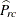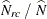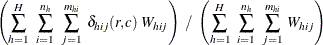If you request BRR variance estimation (by specifying the VARMETHOD=BRR option in the PROC SURVEYFREQ statement), the procedure estimates the variances of proportion estimates as described in the section Balanced Repeated Replication (BRR). If you request jackknife variance estimation (by specifying the VARMETHOD=JACKKNIFE option), the procedure estimates the variances as described in the section The Jackknife Method.

If you do not specify the VARMETHOD= option or a REPWEIGHTS statement, the default variance estimation method is Taylor series, which you can also request with the VARMETHOD=TAYLOR option. By using Taylor series linearization, the variance of a proportion estimate can be expressed as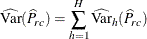where if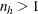,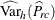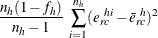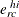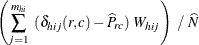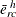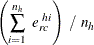and if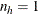,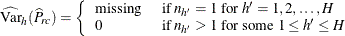The standard error of the proportion is computed as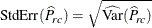Similarly, the estimate of the proportion in row r is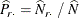And its variance estimate is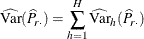where if,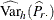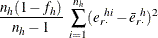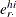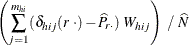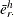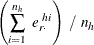and if,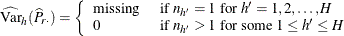The standard error of the proportion in row r is computed as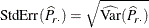Computations for the proportion in column c are done in the same way.